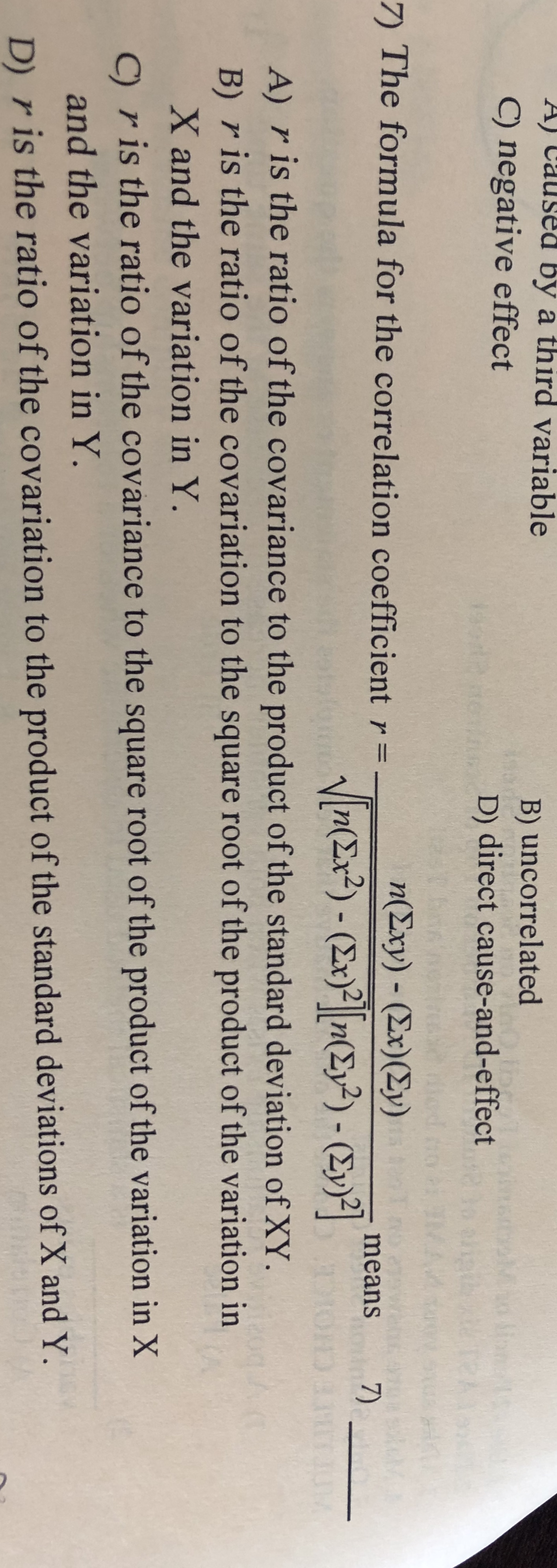# A) Caused by a third variableB) uncorrelated) negative effect0.fb/orrrteD) direct cause-and-effect7) The formula for the correlation coefficient r=n (Σxy)-(Σx) (Σν)means7)n(Ex2)- (Ex)2n(2y2)- (2y)DA) r is the ratio of the covariance to the product of the standard deviation of XYB) r is the ratio of the covariation to the square root of the product of the variation inX and the variation in Y.C)ris the ratio of the covariance to the square root of the product of the variation in Xand the variation in Y.D) r is the ratio of the covariation to the product of the standard deviations of X and Y.

Question
1 viewshelp_outlineImage TranscriptioncloseA) Caused by a third variable B) uncorrelated ) negative effect 0.fb/ orrrte D) direct cause-and-effect 7) The formula for the correlation coefficient r= n (Σxy)-(Σx) (Σν) means 7) n(Ex2)- (Ex)2n(2y2)- (2y) D A) r is the ratio of the covariance to the product of the standard deviation of XY B) r is the ratio of the covariation to the square root of the product of the variation in X and the variation in Y. C)ris the ratio of the covariance to the square root of the product of the variation in X and the variation in Y. D) r is the ratio of the covariation to the product of the standard deviations of X and Y. fullscreen
check_circle

Step 1

The correlation coefficient formula is given by

Step 2

The correlation coefficient is determined by dividing the cova...

### Want to see the full answer?

See Solution

#### Want to see this answer and more?

Solutions are written by subject experts who are available 24/7. Questions are typically answered within 1 hour.*

See Solution
*Response times may vary by subject and question.
Tagged in

### Statistics